Courses

# Chemical Kinetics (Chapter Test - Medical)

## 20 Questions MCQ Test | Chemical Kinetics (Chapter Test - Medical)

Description
This mock test of Chemical Kinetics (Chapter Test - Medical) for Class 12 helps you for every Class 12 entrance exam. This contains 20 Multiple Choice Questions for Class 12 Chemical Kinetics (Chapter Test - Medical) (mcq) to study with solutions a complete question bank. The solved questions answers in this Chemical Kinetics (Chapter Test - Medical) quiz give you a good mix of easy questions and tough questions. Class 12 students definitely take this Chemical Kinetics (Chapter Test - Medical) exercise for a better result in the exam. You can find other Chemical Kinetics (Chapter Test - Medical) extra questions, long questions & short questions for Class 12 on EduRev as well by searching above.
QUESTION: 1

Solution:
QUESTION: 2

Solution:
QUESTION: 3

### For a reaction, A + B→ Products, it is observed that doubling the concentration of B causes the reaction rate to increases four times, but doubling the concentration of A has no effect on the rate of reaction. The rate equation is therefore

Solution:
QUESTION: 4

The following data pertains to reaction between A and
B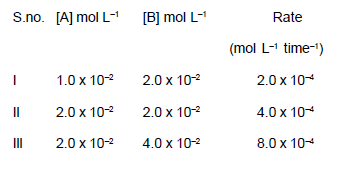Which of the following reference(s) can be drawn from the above data ?
I. Rate constant of the reaction is 1.0 x 10-4
II. Rate law of the reaction is : rate = k [A] [B]
III. Rate of reaction increases four times on
doubling the concentration of both the reactants

Select the correct answer using the codes given below

Solution:
QUESTION: 5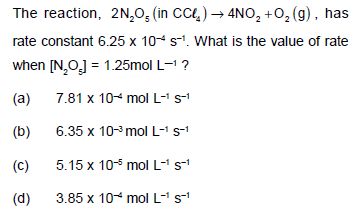Solution: This is decomposition reaction and decomposition reaction is 1order so ROR= K[N2O5]^1 = 6.25×10^-4× 1.25= 7.81×10^-4 ( 1option) is right..
QUESTION: 6

Which one of the following equation is correct for the reaction,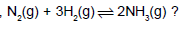Solution:
QUESTION: 7

Units of specific reaction rate for 2nd order reaction is

Solution:
QUESTION: 8

The units of rate constant and rate of reaction are identical for

Solution:
QUESTION: 9

Fill in the blanks in the following table for the reaction X + Y → Z . The reaction is of the first order w.r.t. X and
zero order w.r.t. Y.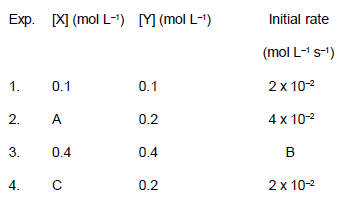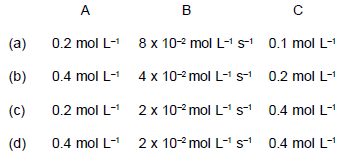Solution:
QUESTION: 10

For a reaction, P +Q → 2R + S . Which of the following
statements is incorrect ?

Solution:
QUESTION: 11

Match the rate law given in column I with the dimensions of rate constants given in column II and mark the
appropriate choice.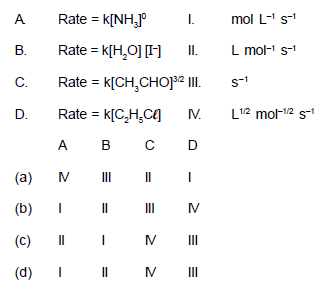Solution:
QUESTION: 12

At room temperature, the reaction between NO and O2 to give NO2 is fast, while that between CO and O2 is
slow. It is due to

Solution:
QUESTION: 13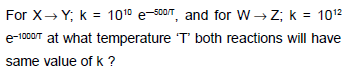Solution:
QUESTION: 14

Which one is not correct ?

Solution:
QUESTION: 15

The rate of equation for the reaction 2A + B→C is found to be : rate = k[A][B]. The correct statement in relation to this reaction is that

Solution:
QUESTION: 16

A mixture of hydrogen and oxygen gases are indefinitely stable at room temperature but if stuck by a spark the mixture immediately explodes because

Solution:
QUESTION: 17

The activation energy for the forward reaction A + B ⇔ C + D is 38 kJ and enthalpy of reaction is 20 kJ. The
activation energy for this same reaction in the reverse direction will be

Solution:
QUESTION: 18

Which of the following simple reactions is trimolecular reaction ?

Solution:
QUESTION: 19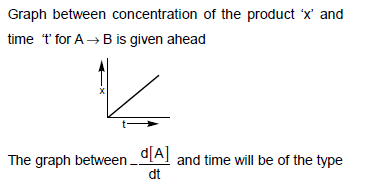Solution:
QUESTION: 20

The half-life period of radioactive materials is 15 minutes. What percent of radioactive of that material
with remain after 45 minutes ?

Solution: